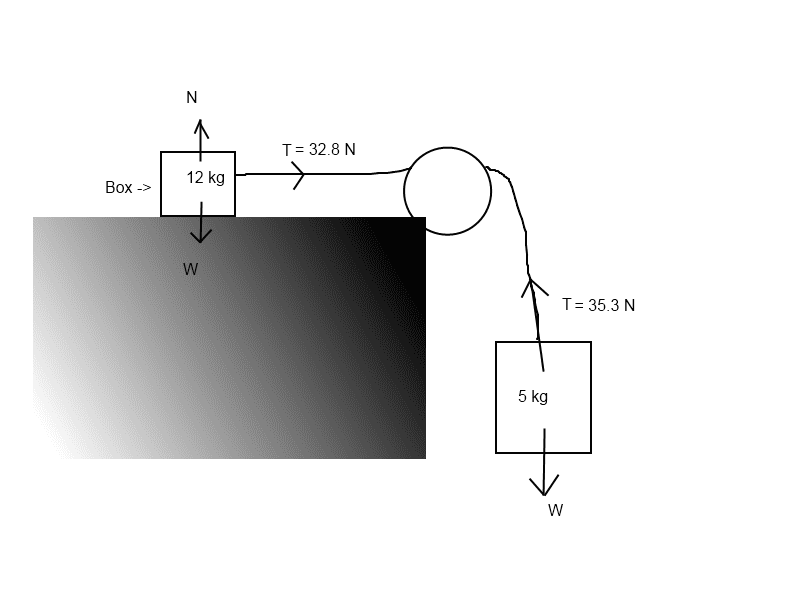# Tension Change in a Wire

tey

## Homework Statement

A 12kg box resting on a horizontal, frictionless surface is attached to a 5kg weight (hanging off the side of the table) by a thin, light wire that passes over a frictionless pulley. The pulley has a shape of a uniform solid disk of mass 2kg and a diameter of .5m. After the system is released, find the tension in the wire on both sides of the pulley

The answer gives different tensions in the wire on either side of the pulley. How is that possible?

## Homework Equations

F = ma, torque = I*angular_acceleration

## The Attempt at a Solution

(a) Tension between 12kg box and pulley = 32.7N, Tension between pulley and 5kg weight is 35.4N.

Homework Helper
Gold Member

## Homework Statement

A 12kg box resting on a horizontal, frictionless surface is attached to a 5kg weight (hanging off the side of the table) by a thin, light wire that passes over a frictionless pulley. The pulley has a shape of a uniform solid disk of mass 2kg and a diameter of .5m. After the system is released, find the tension in the wire on both sides of the pulley

The answer gives different tensions in the wire on either side of the pulley. How is that possible?
For an 'ideal' pulley of negligible friction and mass, the wire tension is the same on both sides of the pulley. Not so for a pulley with mass, because there must be a net torque acting on it to provide it with angular acceleration, per you relevant equation. below.

## Homework Equations

F = ma, torque = I*angular_acceleration

## The Attempt at a Solution

(a) Tension between 12kg box and pulley = 32.7N, Tension between pulley and 5kg weight is 35.4N.
Please show an attempt at a solution using free body diagrams.

Snoogx
I am also having problems understanding this. There is also more parts to the question.

(a) Tensions were correct.

(b) what is the acceleration of the box, after the system is released.

(c)After the system is released, find magnitude of the horizontal and vertical components of the force that the axle exerts on the pulley.

Diagram:Other information:
I = .036
torque = 8.82 N

I really don't know where to start.